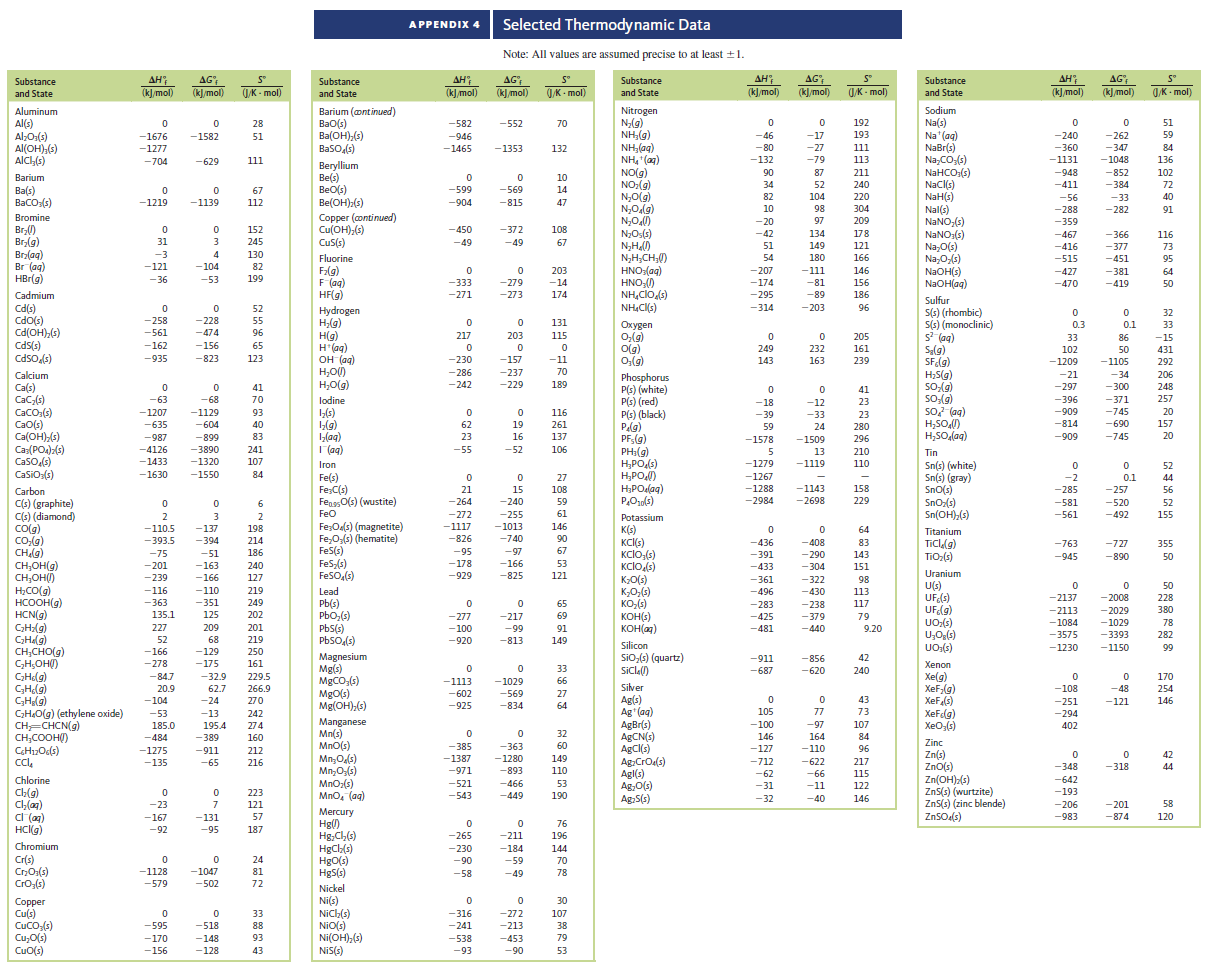# Problem: For the reactionSF4 (g) + F2 (g) → SF6 (g)the value of ΔG° is -374 kJ. Use this value and data from Appendix 4 to calculate the value of ΔG°f for SF4(g).

###### FREE Expert Solution

We are being asked to determine the value of ΔG°f for SF4(g) given the reaction

SF4 (g) + F2 (g)  SF6 (g)

with the value of ΔG° equal to  -374 kJ

Recall the equation for ΔG˚rxn:

81% (89 ratings)###### Problem Details

For the reaction

SF4 (g) + F2 (g) → SF6 (g)

the value of ΔG° is -374 kJ. Use this value and data from Appendix 4 to calculate the value of ΔG°f for SF4(g).Frequently Asked Questions

What scientific concept do you need to know in order to solve this problem?

Our tutors have indicated that to solve this problem you will need to apply the Gibbs Free Energy concept. You can view video lessons to learn Gibbs Free Energy. Or if you need more Gibbs Free Energy practice, you can also practice Gibbs Free Energy practice problems.

What professor is this problem relevant for?

Based on our data, we think this problem is relevant for Professor Keller's class at MIZZOU.

What textbook is this problem found in?

Our data indicates that this problem or a close variation was asked in Chemistry: An Atoms First Approach - Zumdahl Atoms 1st 2nd Edition. You can also practice Chemistry: An Atoms First Approach - Zumdahl Atoms 1st 2nd Edition practice problems.